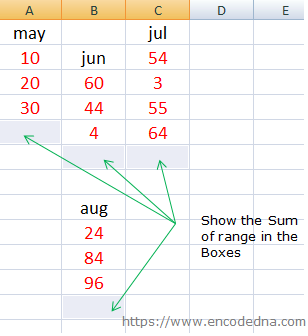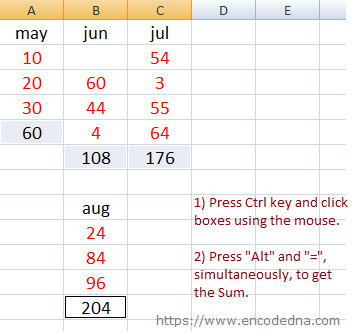﻿ How to AutoSum Multiple Ranges in Excel using ALT Key and Multi-Select

# How to AutoSum Multiple Ranges in Excel using ALT Key and Multi-Select

Shortcut keys are useful when you are working with Ms-Excel or any other application. Using a combination of keyboard shortcuts, you can quickly execute a function or insert a table etc. Here in this post, I’ll show you how to AutoSum multiple ranges in Excel using multi-select along with the Alt key.

## A Scenario

Here’s a simple scenario. I have an Excel worksheet with multiple figures for various Months, scattered all over the sheet. I wish to see the sum of all the figures or values below each month with the press of few buttons, without manually writing the =SUM() function below the figures. See the image below.The arrows in the image shows where I want to show the sum of the figures.

## How do I do it?

We will first multi-select the boxes below each month’s figures.

1) To multi-select boxes, hold the Ctrl key and click each box (where you wish to show the sum) using the mouse. (Ctrl + Click)
2) Once you have selected the boxes, leave the Ctrl key the press Alt and = keys simultaneously. (Alt =)

Also Read: How to Find Duplicates in Excel using Conditional Formating

The Alt= key combination will automatically add the =sum() function below each months figures, along with a range. For example, for the first column in the above example, the range would be =SUM(A2:A4).

### Output